Every student is a promise

# Understanding the Progress Report with/without the Weight Grades

A progress report shows a detailed progress performance with each assignment in a quarter marking period. The report may vary depending on the weight grades if they are switched on from the configuration setup or not.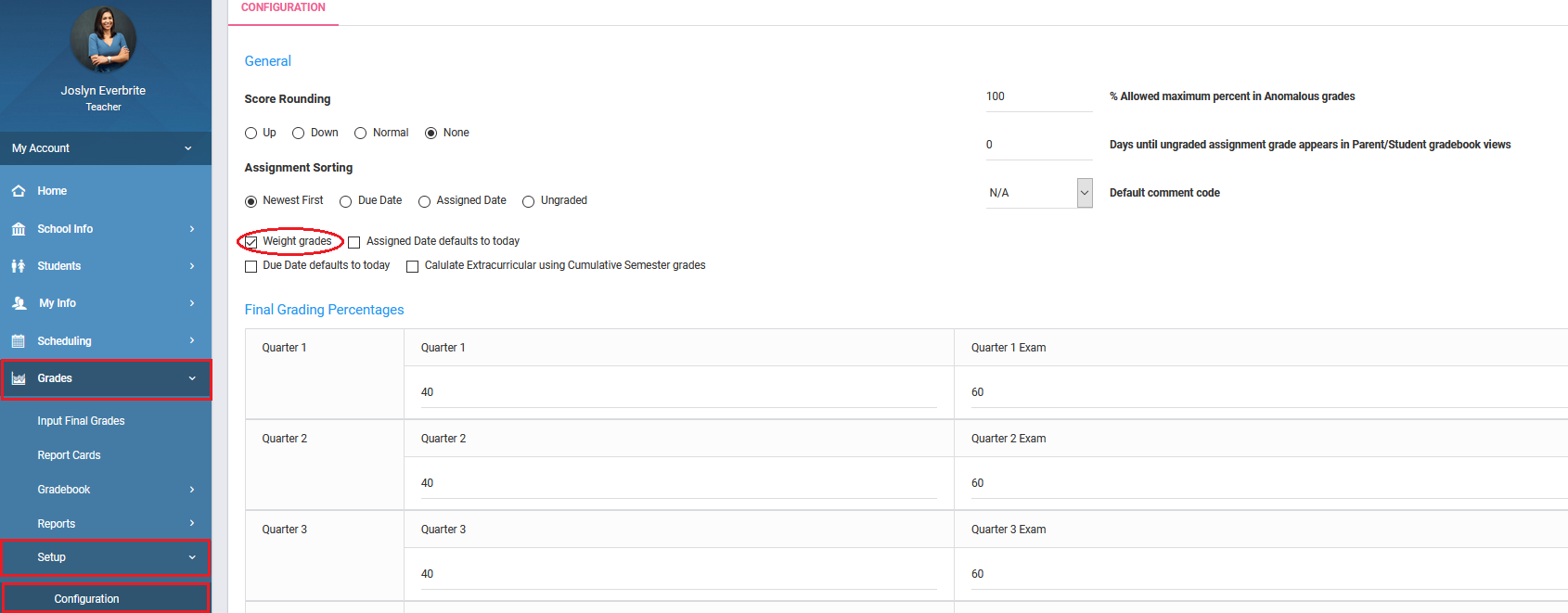With Weight Percentage

If the weight grades is switched on, make sure the weight percentage is added to the assignment types.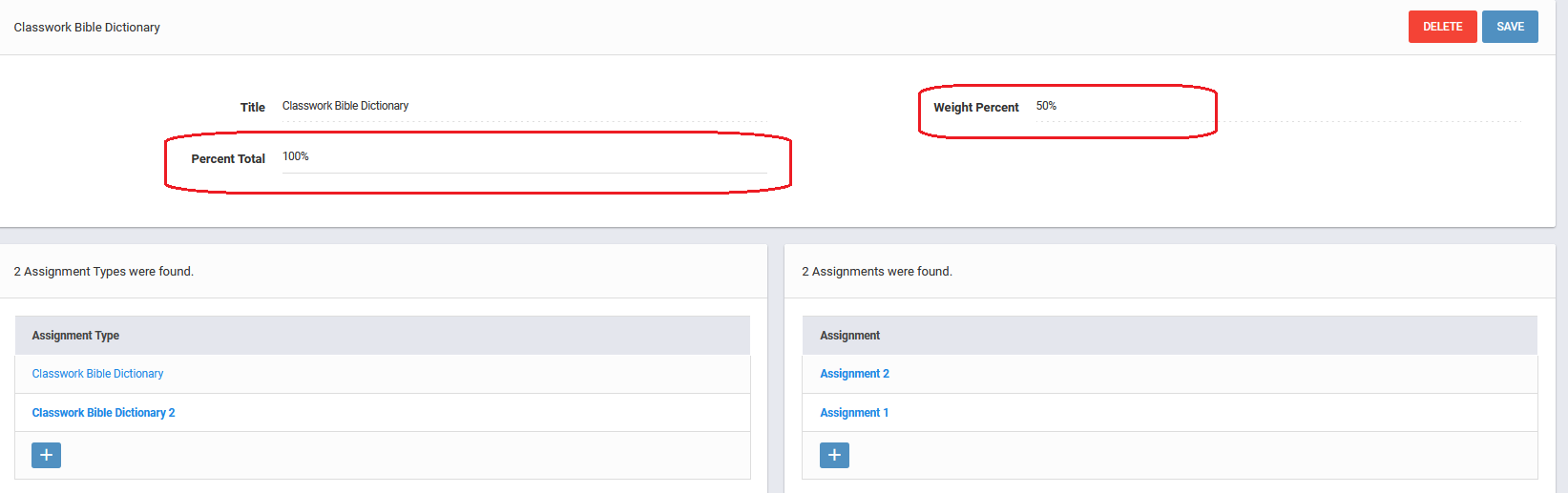Now, after the grades are entered in respect with these assignments, the progress reports shows the following calculation.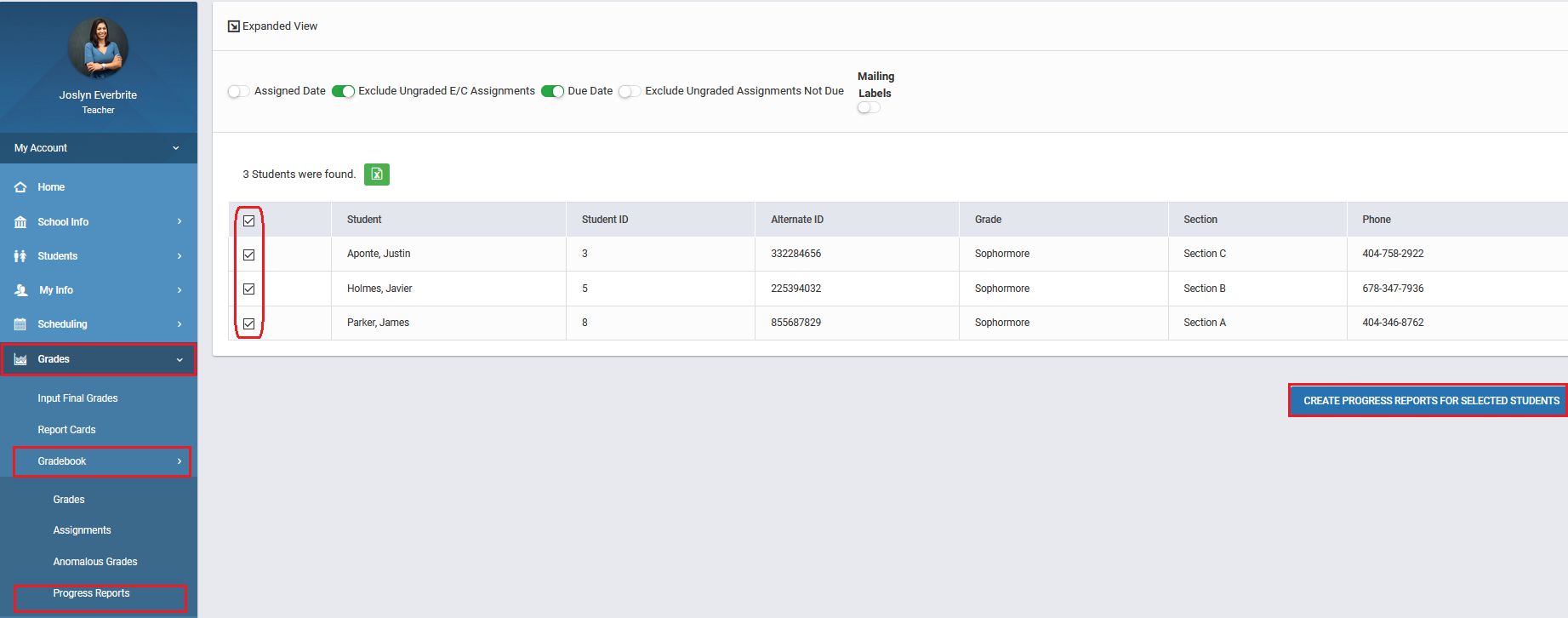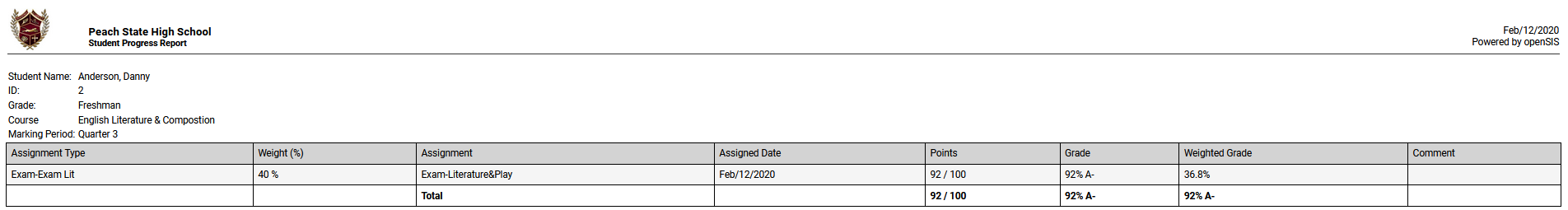The total shows 88.8% which is

Classwork Type (weight % is 60%)

Weighted Grade of Assignment 1 – 60% of 86 = 51.6%

Weighted Grade of Assignment 2 – 60% of 82 = 49.2%

Now, Average of the weighted grades in the same assignment type, i.e. (51.6 + 49.2) / 2 = 100.8/2 or, 50.4

Homework Type (Weight % is 40%)

Weighted Grade of Assignment 3 – 40% of 96 = 38.4%

Now, Average of the weighted grades in the same assignment type, i.e. 38.4 / 1 = 38.4

And, the TOTAL value will be 50.4 + 38.4 = 88.8%

Thus, this is the final value after weight percentage.

Without Weight Percentage

If the weight grades is switched off, the weight percentage field will not appear in the assignment type.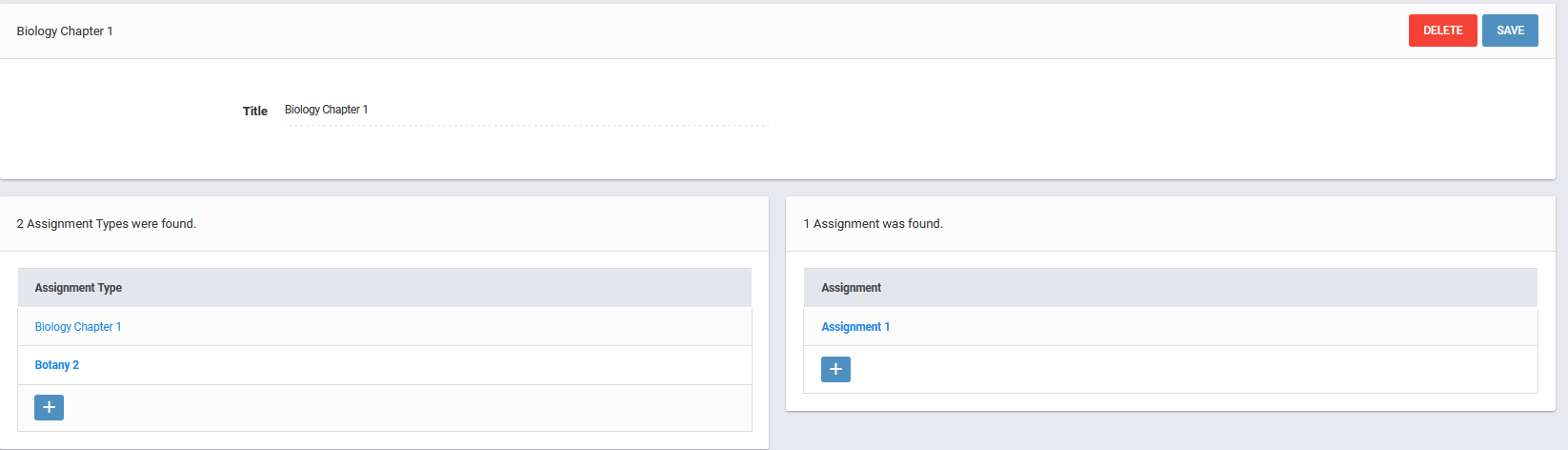Now, after the grades are entered in respect with these assignments, the progress reports shows the following calculation.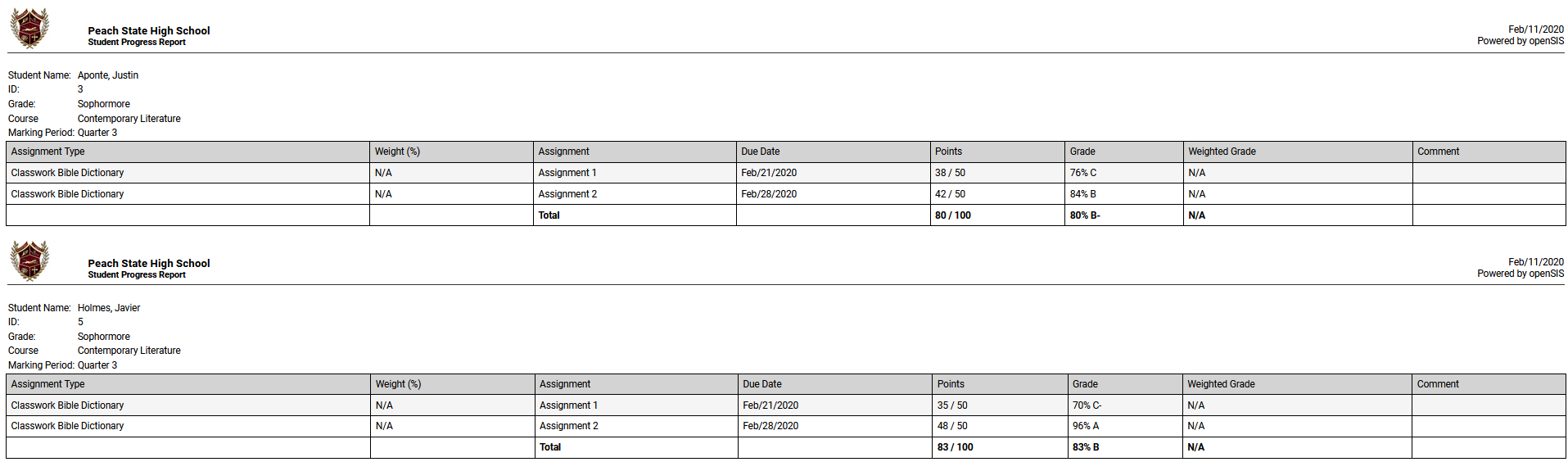The total shows 88% which is

132/150 = 88% [all the earned points over the total points of the assignments, i.e. (43+41+48) / (50+50+50)]

Thus, this is the final value without weight percentage.